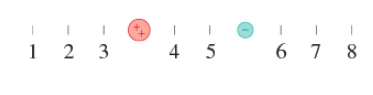# Problem: A positive and negative charge are shown in (Figure 1) .At which numbered position (or positions) is the electric potential zero?

###### FREE Expert Solution

Considering the negative charge at a point x, the distance between the positive charge +2q and the point is 3 - x. This is where the electric potential is zero.

82% (3 ratings)###### Problem Details

A positive and negative charge are shown in (Figure 1) .At which numbered position (or positions) is the electric potential zero?